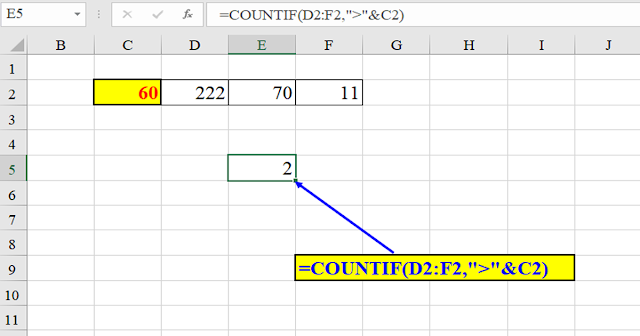## יום ראשון, 24 באוקטובר 2021

### Excel - SUM instead of….COUNTIF

Consider the following case:

You have a number in cell C2.

The adjacent cells: D2:F2 also contain numbers.

The challenge: you want to find how many numbers in Cells D2:F2 are greater than the number in C2.

The most obvious solution would be with the COUNTIF function:

=COUNTIF(D2:F3,”>”&C2)Picture 1: The COUNTIF solution

However, a simpler, shorter solution would be by using the SUM function:

=SUM(1*(D2:F2>C2))

Picture 2: The SUM solution

The (D2:F2>C2) part of the formula returns a list of TRUEs and FALSEs.

In order to convert them into numbers, we multiply the comparison’s result by 1.

So, instead of the TRUE, TRUE, FALSE we have a 3-member array: 1,1,0

Now the SUM functions adds them all together: 1+1+0 which yields the result: 2.

P.s.
This solution uses Excel 365.
Since this is an array formula, if you're using an earlier version, (2013,2016,2019...) you should press: CTRL+SHIFT+ENTER instead of: ENTER, when you end typing the formula.

That’s all.

I hope you found this “trick” useful.